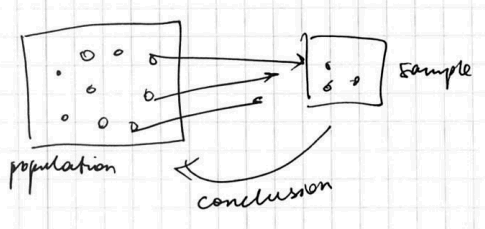# ML Wiki

## Sampling

Statistical Inference - making conclusions and decisions incomplete information in based on data. This is the main goal of Statistics

• Population - the group we're interested in making conclusions about.
• Census - collection of data from the entire population
• Census is almost impossible or very expensive to obtain
• Sample - a subset of the population, typically a small fraction

### Goals

So, the goal of sampling (data collection):

• based on a sample make conclusions about the population
• this is done at the Data Collection step in the process of statistical investigation (see Statistics)For ML models there are other goals

• how to reduce data to speed up computation?
• select a subset of rows - a sample

## Types of Sampling

We need a representative sample to be able to generalize from the statistics calculated on a sample to the population parameters

## Random Sampling

Random sampling (especially SRS - simple random sampling) is very important

• in Inferential Statistics - when making the independence assumption about the observations
• doesn't introduce bias

### Replacements

• without replacement
• when item is selected for a sampling, it's taken out of the population
• sampling with replacement
• an item can be sampled several times
• used in the Bootstrap method - for resampling
• also see Simulation Basics in R#Sampling

### Simple Random Sampling

Randomly pick up items from the population

### Stratified Sampling

Stratified Sampling

• divide the population into non-overlapping groups (called strata)
• and use SRS within each stratum
• so the original distribution is kept

Also called

• Sampling with proportional allocation
• Under-sampling of the majority class
• etc

### Cluster Sampling

Cluster Sampling

• use Cluster Analysis to divide the population into clusters
• select a cluster at random and use all the items from that cluster
• Use then it's easer to select a group than an item

### Examples

#### Example 1

• 1 mln elements
• 5% True, 95% False
• want to sample 100 examples
• proportional (stratified): 5 True, 95 False
• without proportional (uniform): 50 True, 50 False

Reason to use proportional

• suppose you need to be good at detecting TRUE
• but you'll have only 5 records to train your classified - not enough!
• so it's better to use stratified sampling

#### Stratified Sampling Example

Assume a company with the following allocation of staff

Full Time Part Time
Male 90 18
Female 9 63

How to build a sample of 40 staff?

• Stratified with proportional allocation: according to the distribution
• total number: $N = 180$
• calculate the percentage in each group
Full Time Part Time
Male 90 / 180 = 50% 18 / 180 = 10%
Female 9 / 180 = 5% 63 / 180 = 35%

So we know that

• 50% in out sample of 40 should be males, full time
Full Time Part Time
Male 20 4
Female 2 14

## Non-Random Sampling

• Systematic sampling
• every $n$th individual, non-representative is there's a structure
• Convenience / Volunteer sampling
• select first $n$ who are available or volunteer to participate. Also non-representative
• all these may introduce bias into the samples

## Bias

• A sample is biased if it's differs from a population in a systematic way
• That can result in a statistics that's consistently larger or smaller

### Types of Biases

• Selection bias - when you systematically exclude or under-represent a part of population
• Measurement/Response bias - when data is collected with systematic error
• Non-response bias - when responses aren't obtained from all individuals selected for inclusion in sampling